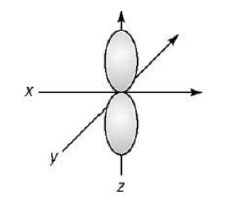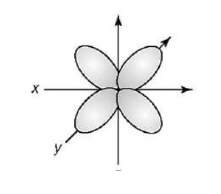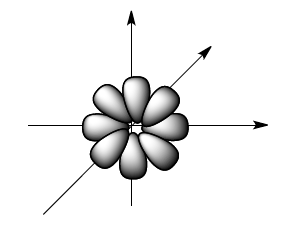Ch.7 - Quantum MechanicsWorksheetSee all chapters
 Ch.1 - Intro to General Chemistry 4hrs & 4mins 0% complete Worksheet Ch.2 - Atoms & Elements 4hrs & 21mins 0% complete Worksheet Ch.3 - Chemical Reactions 4hrs & 18mins 0% complete Worksheet BONUS: Lab Techniques and Procedures 1hr & 38mins 0% complete Worksheet BONUS: Mathematical Operations and Functions 47mins 0% complete Worksheet Ch.4 - Chemical Quantities & Aqueous Reactions 3hrs & 54mins 0% complete Worksheet Ch.5 - Gases 3hrs & 22mins 0% complete Worksheet Ch.6 - Thermochemistry 2hrs & 26mins 0% complete Worksheet Ch.7 - Quantum Mechanics 2hrs & 17mins 0% complete Worksheet Ch.8 - Periodic Properties of the Elements 2hrs & 43mins 0% complete Worksheet Ch.9 - Bonding & Molecular Structure 2hrs & 52mins 0% complete Worksheet Ch.10 - Molecular Shapes & Valence Bond Theory 1hr & 31mins 0% complete Worksheet Ch.11 - Liquids, Solids & Intermolecular Forces 3hrs & 40mins 0% complete Worksheet Ch.12 - Solutions 2hrs & 17mins 0% complete Worksheet Ch.13 - Chemical Kinetics 2hrs & 23mins 0% complete Worksheet Ch.14 - Chemical Equilibrium 2hrs & 26mins 0% complete Worksheet Ch.15 - Acid and Base Equilibrium 4hrs & 42mins 0% complete Worksheet Ch.16 - Aqueous Equilibrium 3hrs & 48mins 0% complete Worksheet Ch. 17 - Chemical Thermodynamics 1hr & 44mins 0% complete Worksheet Ch.18 - Electrochemistry 3hrs & 3mins 0% complete Worksheet Ch.19 - Nuclear Chemistry 1hr & 31mins 0% complete Worksheet Ch.20 - Organic Chemistry 3hrs 0% complete Worksheet Ch.22 - Chemistry of the Nonmetals 2hrs & 1min 0% complete Worksheet Ch.23 - Transition Metals and Coordination Compounds 1hr & 54mins 0% complete Worksheet

# Quantum Mechanics Problems

See all sections
Sections
Wavelength and Frequency
Speed of Light
The Energy of Light
Electromagnetic Spectrum
Photoelectric Effect
De Broglie Wavelength
Heisenberg Uncertainty Principle
Bohr Model
Emission Spectrum
Bohr Equation
Introduction to Quantum Mechanics
Quantum Numbers: Principal Quantum Number
Quantum Numbers: Angular Momentum Quantum Number
Quantum Numbers: Magnetic Quantum Number
Quantum Numbers: Spin Quantum Number
Quantum Numbers: Number of Electrons
Quantum Numbers: Nodes
Diffraction vs Refraction
Quantum Numbers: Emission Spectrum
Dimensional Boxes

Test your skills on the following problems.

###### Emission vs. Absorption

Example #1: Which of the following transitions (in a hydrogen atom) represent emission of the smallest or shortest wavelength?

a)  n = 4 to n = 2

b)  n = 3 to n= 4

c)  n = 1 to n = 2

d)  n = 7 to n = 5

e)  n = 2 to n = 5

Example #2: Which of the following transitions represent absorption of a photon with the highest frequency?

a)  n = 3 to n = 1

b)  n = 2 to n = 4

c)  n = 1 to n =2

d)  n = 6 to n = 3

e)  n = 1 to n = 3

###### Quantum Numbers

Example #3: Provide the n, l and ml value for each of the given orbitals.

a) 7s      n =  ?                             b) 5d     n =  ?

l  =   ?                                         l =  ?

ml =   ?                                        ml =  ?

c) 2p     n =    ?                            d) 4f      n =  ?

l  =   ?                                          l  =   ?

ml =     ?                                      ml =  ?

Example #4: Which statement about the four quantum numbers is false?

a)  n = principal quantum number,            n = 1 to infinity

b)  l = azimuthal quantum number,           l = 0,1,2, . . ., (n+1)

c)  mL = magnetic quantum number, mL = (-l), . . .,0,. . ., (+l)

d)  ms = spin quantum number,    ms = + 1/2  or − 1/2

e)  The first three quantum numbers deal with the atomic orbitals except for the ms quantum   number, which deals with the electrons in the atomic orbitals.

Example #5: Each of the following sets of quantum numbers gives information on a specific orbital. Find the error in each.

a. n = 4, l = 0 , ml = 1, ms = – 1/2

b. n = 5, l = 2 , ml = - 1, ms = 1

c. n = 7, l = 7, ml = - 5, ms = – 1/2

d. n = 0, l = 5, ml = - 3, ms = 1/2

Example #6: How many electrons can have the following quantum sets?

a) n = 4, mL = -1

b) n = 5, mL = 0 , mS = – 1/2

c) n = 9, l = 4, mS = – 1/2

d) n = 2, mS = 1/2

Example #7: For n = 2, what are the possible sublevels?

a) 0

b) 0, 1

c) 0, 1, 2

d) 0, 1,2, 3

###### Quantum Numbers & Atomic ShapeExample #8: Based on the following atomic orbital shape, which of the following set of quantum numbers is correct:

a) n = 2, l = 1, ml = 0

b) n = 3, l = 2, ml = –1

c) n = 4, l = 0, ml = +1

d) n = 1, l = 1, ml = 0Example #9: Based on the following atomic orbital shape, which of the following set of quantum numbers is correct:

a) n = 3, l = 2, ml = 0, ms = – 1/2

b) n = 3, l = 1, ml = – 3, ms = 1

c) n = 4, l = 0, ml = 0, ms = – 1/2

d) n = 4, l = 2, ml = – 3, ms = 1/2Example #10: Based on the following atomic orbital shape, which of the following set of quantum numbers is correct:

a) n = 3, l = 3, ml = 0, ms = 1/2

b) n = 1, l = 3, ml = – 3, ms = 1

c) n = 7, l = 3, ml = – 4, ms = – 1/2

d) n = 6, l = 3, ml = – 3, ms = – 1/2

###### Paramagnetism vs. Diamagnetism

Example #11: Give the electron configuration for the following element and its ion. For the ion, state if it is paramagnetic or   diamagnetic:

Ag and Ag+

Example #12: Give the electron configuration for the following element and its ion. For the ion, state if it is paramagnetic or   diamagnetic:

Cl and Cl+

###### Electron Configurations

Example #13: Which of the following represents an “excited” state?

a) Cl: 1s22s22p63s23p5

b) Be: 1s22s2

c) Na: 1s22s22p63p1

d) N: 1s22s22p3

Example #14: Give the set of four quantum numbers that represent the indicated electron in the following element:

a. Br (33rd electron)      n = ,        l = ,        ml = ,        ms =

Example #15: Give the set of four quantum numbers that represent the indicated electron in the following element:

a. Ca (19th electron)         n = ,        l = ,          ml = ,             ms =

Example #16: Give the set of four quantum numbers that represent the indicated electron in the following element:

a. Cu (27th electron)        n = ,         l = ,        ml = ,            ms =

Example #17: Give the set of four quantum numbers that represent the indicated electron in the following element:

a. Mo3+ (38th electron)       n = ,         l = ,       ml = ,         ms =

Example #18: For a multi-electron atom, arrange the electron subshells of the following listing in order of increasing energy:

6s, 4f, 2p, 5d.